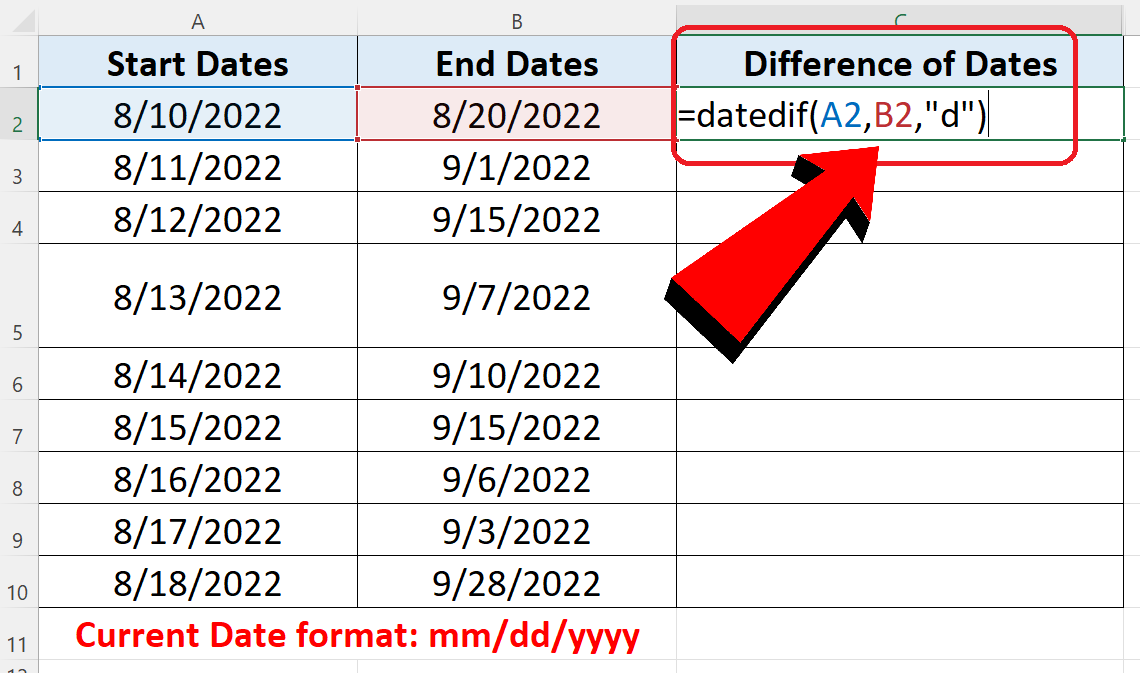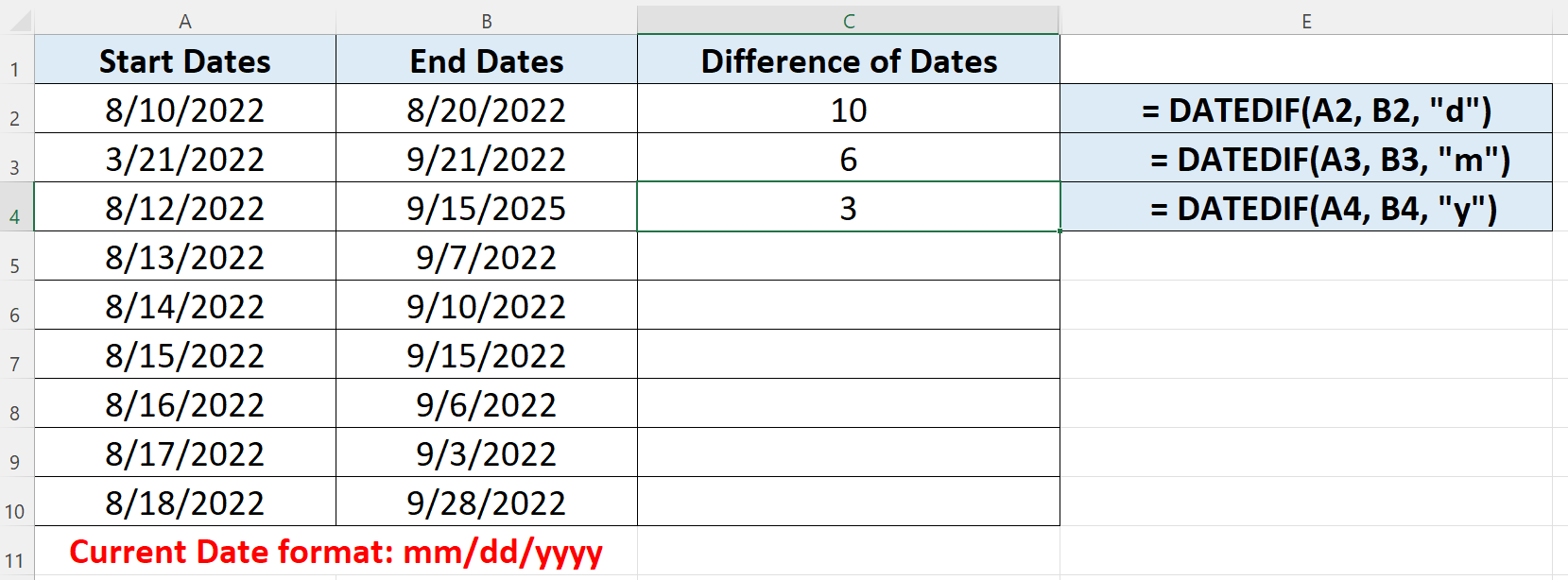# How to count date difference in Excel

Excel has the built-in function to calculate the date difference through the “DATEDIF” function. The best way to understand how DATEDIF works is to first understand the structure of the function and the parameters it requires.

## Structure of DATEDIF

The standard syntax of DATEDIF function in excel is shown below;

=DATEDIF(start_date, end_date, unit)

• start_date

Could be any date earlier than the second parameter end date. Otherwise errors will occur.

• end_date

Could be any date later than the first parameter start date. Otherwise errors will occur.

• unit

Could be any one from “d” for days, “m” for months and “y” for years.

Implementing the Formula on an Actual Data Set

Let’s take an example data set and find out the number of days between some actual dates. So let’s consider the data set shown above;

We can see in the example data set that there are some dates and start dates that are earlier than the end dates.

Let’s see how we can use Excel’s built-in formula to find a solution to our problem by following these steps;Sometimes we encounter the problem of counting the difference of various dates while working in Excel. One such situation can happen when you have to track the days your team worked on a particular task. So logging all dates in excel can help you in a magical way and you can calculate the difference of dates very easily.

Let’s see how we can use Excel’s built-in formula to find a solution to our problem by following these steps;

### Step 1 – Implement the formula to calculate Days Difference– Choose a suitable cell where you wish to implement the formula. In our example it is C2.

– Use the following formula in that cell and press enter key.
= DATEDIF(A2:B2,”d”)

– The difference of dates will be displayed in this cell as soon as you press the enter key as shown in the picture above.

So by using DATEDIF we calculated the difference of the first date. We can drag down the formula to extend it to the whole data set. However, there are some useful variations of this formula which are discussed in the next steps.

### Step 2 – Implement the formula to calculate Months or Years Difference– Now we will use the same formula to calculate the difference of months and years between two dates. For this go to any cell, let’s say C3.

– Use the following formula in that cell to calculate the difference in months between two dates and press enter key.
= DATEDIF(A3:B3,”m”)

– The difference of months will be displayed in this cell as soon as you press the enter key.

– Similarly we can calculate the difference in years between two dates using the same formula with slight modification. This time use the following formula
= DATEDIF(A4:B4,”y”)

– The results will be displayed in respective cells as shown above.

So by using Excel’s DATEDIF function we can easily find the difference of days, months and years between two dates.